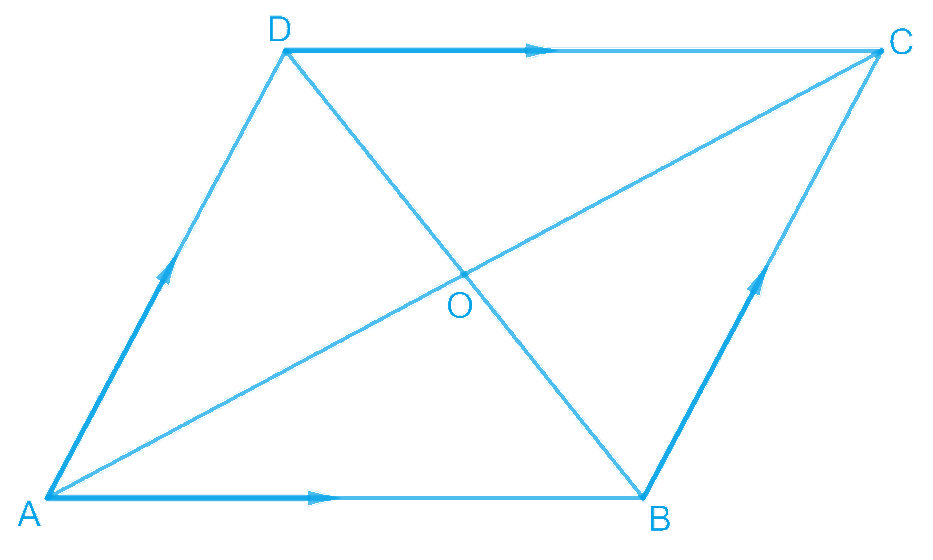# Ex.8.1 Q2 Quadrilaterals Solution - NCERT Maths Class 9

## Question

If the diagonals of a parallelogram are equal, then show that it is a rectangle.

Video Solution
Quadrilaterals
Ex 8.1 | Question 2

## Text Solution

What is known/?

The diagonals of a parallelogram are equal.

What is unknown?

How we can show that it is a rectangle.

Reasoning:

To show that given parallelogram is a rectangle, we have to prove that one of its interior angles is and this can be done by showing two triangles congruent.

Steps:Let $$ABCD$$ be a parallelogram. To show that $$ABCD$$ is a rectangle, we have to prove that one of its interior angles is \begin{align}90^{\circ}\end{align} .

In $$\Delta ABC$$ and  $$\Delta DCB$$

\begin{align} & AB=DC\, \\ & \left(\!\begin{array} & \text{In a parallelogram, } \\ \text{opposite sides are equal}\text{.} \\ \end{array}\!\right) \\ & \\ & BC=BC\,\left( \text{Common} \right) \\ & AC=DB\,\left( \text{Given} \right) \\ & \therefore \Delta ABC\,\cong \,\Delta DCB \\ & \left( By\,SSS\,\text{Congruence rule} \right) \\ & \\ & \Rightarrow \,\,\,\angle ABC=\angle DCB \\ \end{align}

It is known that the sum of the measures of angles on the same side of transversal is $$180^\circ$$.

\begin{align}\angle &{\rm{ABC}} + \angle {\rm{DCB}} = {\rm{18}}{0^0}\;\left( {{\rm{AB }}||{\rm{ CD}}} \right)\\&\Rightarrow {\rm{ }}\angle {\rm{ABC}} + \angle {\rm{ABC}} = {\rm{18}}{0^0}\\&\Rightarrow {\rm{ 2}}\angle {\rm{ABC}} = {\rm{18}}{0^0}\\& \Rightarrow {\rm{ }}\angle {\rm{ABC}} = {\rm{9}}{0^0}{\rm{ }}\end{align}

Since $$ABCD$$ is a parallelogram and one of its interior angles is \begin{align}90^{\circ},\end{align} $$ABCD$$ is a rectangle.

Video Solution
Quadrilaterals
Ex 8.1 | Question 2
Learn from the best math teachers and top your exams

• Live one on one classroom and doubt clearing
• Practice worksheets in and after class for conceptual clarity
• Personalized curriculum to keep up with school